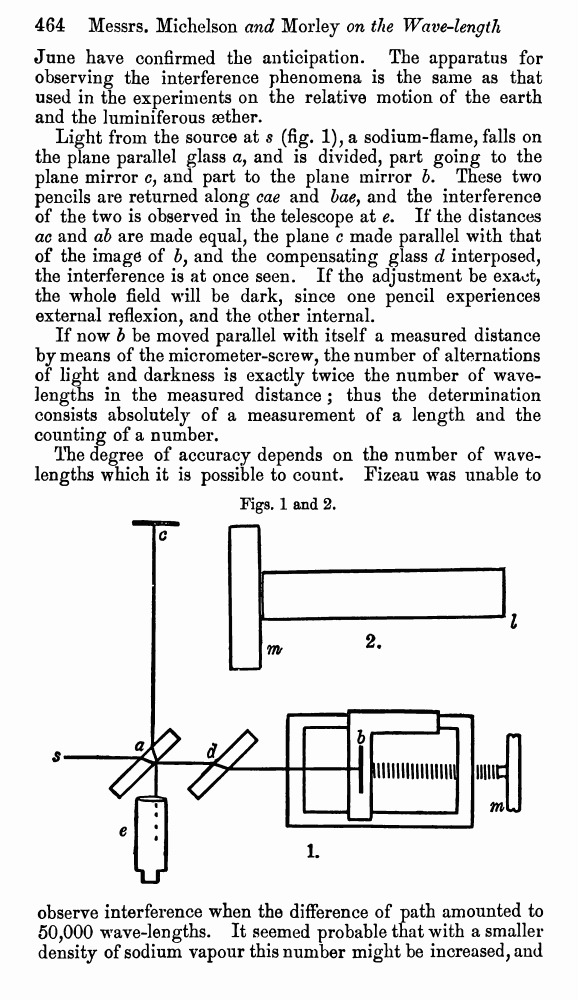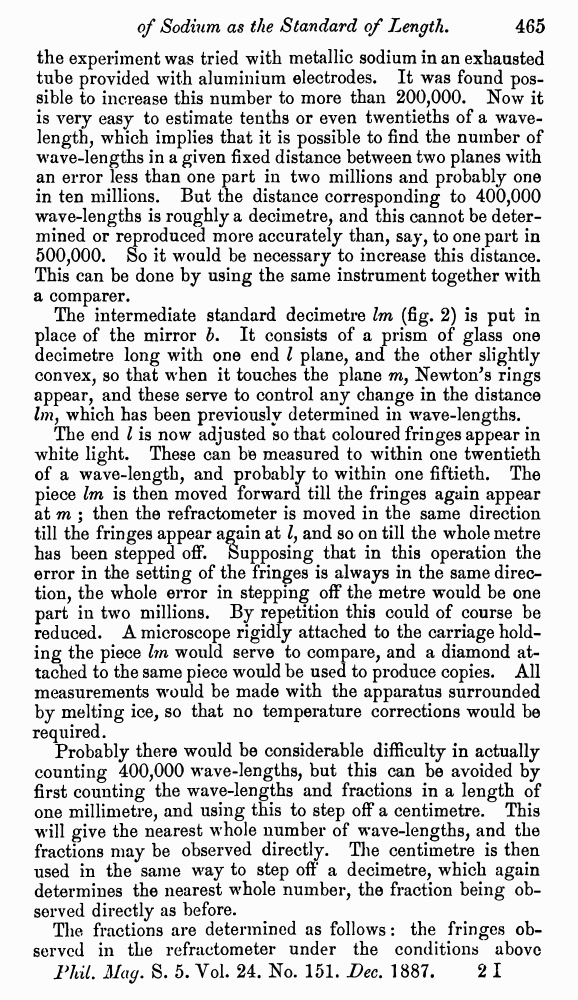# A. Michelson and E. Morley. On the Relative Motion of the Earth and the Luminiferous Æther. // Phil. Mag. S. 5. Vol. 24. No. 151. Dec. 1887.

В начало   Другие форматы   <<<     Страница 464   >>>449  450  451  452  453  454  455  456  457  458  459  460  461  462  463  464 465  466 June have confirmed the anticipation. The apparatus for observing the interference phenomena is the same as that used in the experiments on the relative motion of the earth and the luminiferous æther. Light from the source at s (fig. 1), a sodium-flame, falls on the plane parallel glass a, and is divided, part going to the plane mirror c, and part to the plane mirror b. These two pencils are returned along cae and bae, and the interference of the two is observed in the telescope at e. If the distances ac and ab are made equal, the plane c made parallel with that of the image of b, and the compensating glass d interposed, the interference is at once seen. If the adjustment be exact, the whole field will be dark, since one pencil experiences external reflexion, and the other internal. If now b be moved parallel with itself a measured distance by means of the micrometer-screw, the number of alternations of light and darkness is exactly twice the number of wavelengths in the measured distance ; thus the determination consists absolutely of a measurement of a length and the counting of a number. The degree of accuracy depends on the number of wavelengths which it is possible to count. Fizeau was unable to Figs. 1 and 2. observe interference when the difference of path amounted to 50,000 wave-lengths. It seemed probable that with a smaller density of sodium vapour this number might be increased, and the experiment was tried with metallic sodium in an exhausted tube provided with aluminium electrodes. It was found possible to increase this number to more than 200,000. Now it is very easy to estimate tenths or even twentieths of a wavelength, which implies that it is possible to find the number of wave-lengths in a given fixed distance between two planes with an error less than one part in two millions and probably one in ten millions. But the distance corresponding to 400,000 wave-lengths is roughly a decimetre, and this cannot be determined or reproduced more accurately than, say, to one part in 500,000. So it would be necessary to increase this distance. This can be done by using the same instrument together with a comparer. The intermediate standard decimetre lm (fig. 2) is put in place of the mirror b. It consists of a prism of glass one decimetre long with one end l plane, and the other slightly convex, so that when it touches the plane m, Newton's rings appear, and these serve to control any change in the distance which has been previously determined in wave-lengths. The end l is now adjusted so that coloured fringes appear in white light. These can be measured to within one twentieth of a wave-length, and probably to within one fiftieth. The piece lm is then moved forward till the fringes again appear at m ; then the refractometer is moved in the same direction till the fringes appear again at l, and so on till the whole metre has been stepped off. Supposing that in this operation the error in the setting of the fringes is always in the same direction, the whole error in stepping off the metre would be one part in two millions. By repetition this could of course be reduced. A microscope rigidly attached to the carriage holding the piece lm would serve to compare, and a diamond attached to the same piece would be used to produce copies. All measurements would be made with the apparatus surrounded by melting ice, so that no temperature corrections would be required. Probably there would be considerable difficulty in actually counting 400,000 wave-lengths, but this can be avoided by first counting the wave-lengths and fractions in a length of one millimetre, and using this to step off a centimetre. This will give the nearest whole number of wave-lengths, and the fractions may be observed directly. The centimetre is then used in the same way to step off a decimetre, which again determines the nearest whole number, the fraction being observed directly as before. The fractions are determined as follows : the fringes observed in the refractometer under the conditions above